Navigate back to the homepage

# Functional Programming in Kotlin - f(3)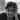Hari Vignesh Jayapalan
May 14th, 2020 · 2 min read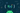## Function Composition

This is the third part of the functional programming series. If you have missed the previous one, start here.

### Series pit stops

In this pit stop, we’ll take a brief look at how to compose functions.

### Pre-requisites

• Basic programming knowledge
• School mathematics
• Previous pit stop

### Mathematical Composition

In our first pit stop, we understood how to compose two function or sets mathematically. To brush up quickly, I’m including a small gist here again. If you are confident, please move to next section (Kotlin Composition).

Functions are like legos, meaning — you can use them to build or compose other functions. In other words, function composition is an operation on functions to produce a new function.

For example,

• Consider function1: f(x) = x + 1
• Consider function2: g(x) = 2x
• Our aim, to compose a function h(x) using f(x) and g(x)

The composition of a function f over g is usually denoted as f o g. This is pronounced as f of g or f round g or can be denoted as f(g(x)). Which gives us,

`.css-1chxjt6{position:absolute;right:22px;top:24px;padding:8px 12px 7px;border-radius:5px;color:#6f7177;-webkit-transition:background 0.3s ease;transition:background 0.3s ease;}.css-1chxjt6:hover{background:rgba(255,255,255,0.07);}.css-1chxjt6[data-a11y="true"]:focus::after{content:"";position:absolute;left:-2%;top:-2%;width:104%;height:104%;border:2px solid var(--theme-ui-colors-accent,#6166DC);border-radius:5px;background:rgba(255,255,255,0.01);}@media (max-width:45.9375em){.css-1chxjt6{display:none;}}1h(x) = f o g = f(g(x) = f(2x) = (2x) + 1 => 2x + 1`

The value of g(x) is substituted in place of x in f(x) ☝️

Hence, f o g = 2x + 1

Fun fact: You can also compose g o f, but f o g and g o f are not equal operations. they may result in the same sometimes though. If we compose g round f for the above functions,

`1g o f = g(f(x)) = 2(x+ 1) = 2x + 2`

Hence, for the mentioned f(x) and g(x), f o g != g o f

#### Pictographic representation

If the equations are confusing for you 👇 (you are not alone 🤫)

`1Given: x = { 1, 2, 3, 4 }`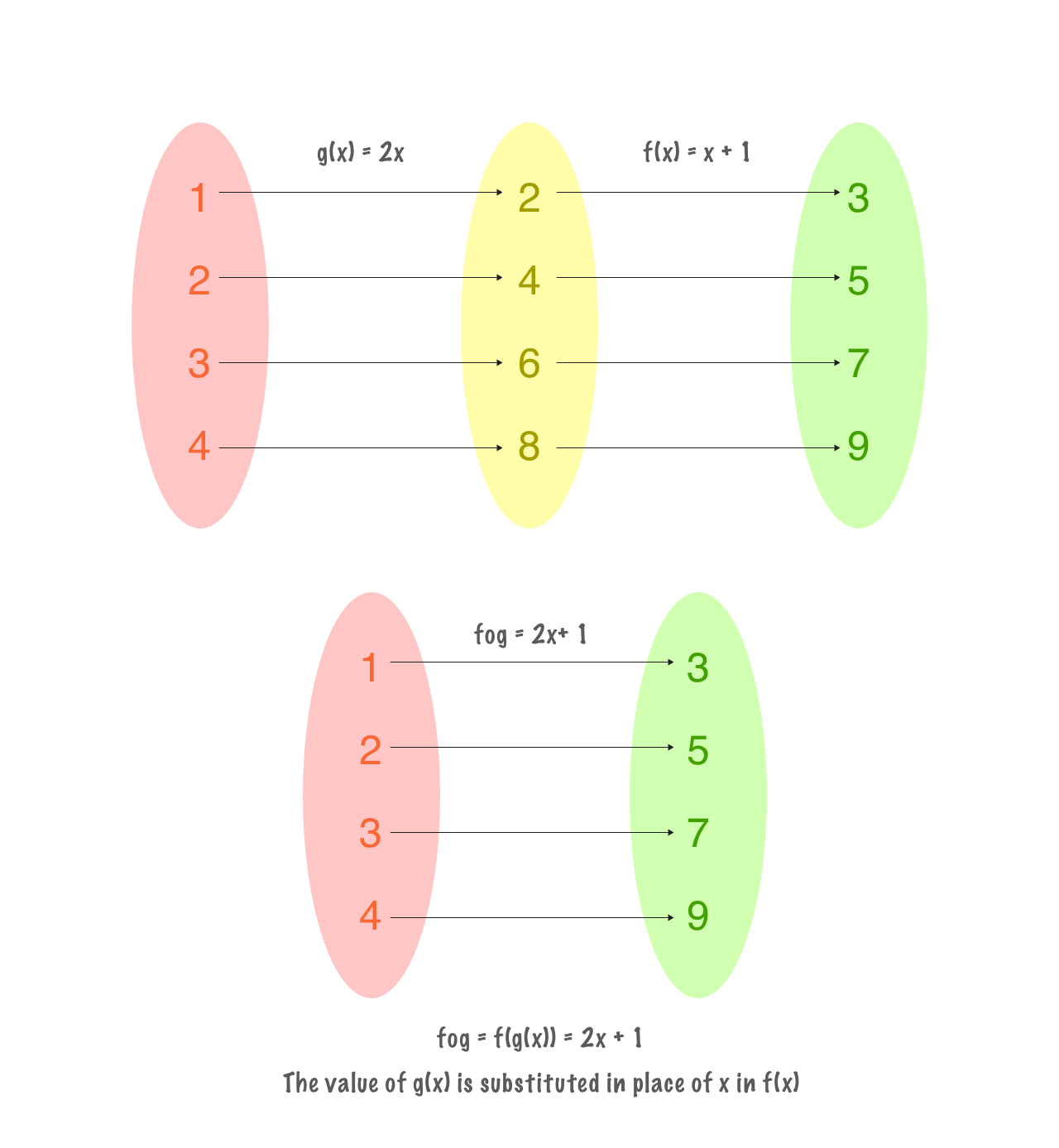### Kotlin Composition

Let’s try to compose the same functions in Kotlin

`1fun f(x: Int) = x + 12fun g(x: Int) = 2 * x3    4println(f(g(2))  // output -> 5`

If you think that’s how the function composition is done using functions, then you are wrong

A function composition is like a binary operation on functions, just like adding integers or numbers, composition is also an operation on functions.

`1fun f(x: Int) = x + 12fun g(x: Int) = 2 * x34// function composition5fun compose(f: (Int) -> Int, g: (Int) -> Int): (Int) -> Int = 6{ x -> f(g(x)) }`

The compose function, takes in two functions as parameter where each of them have an integer as a parameter and as a return value and it also returns a function of integer as a parameter and as a return value.

We now use the compose function to compose f and g

`1val fog = compose(::f, ::g)23println(fog(2)) // output -> 5`

Fun fact: Functions in Kotlin supports functional references (::). We use this operator to access the reference of a function. Just like how we pass value by reference. If the function is of value type or property, then reference is not needed.

### Polymorphic function composition

The compose function that we created is restricted to one type. What if we need to compose a function of generic type?

`1fun r1(x: Boolean): Int = if(x) 1 else 02fun r2(x: Int): Boolean = if(x == 0) false else true34fun <T, U, V>compose(f: (U) -> V, g: (T) -> U): (T) -> V = 5{ f(g(it)) }67val r1of2 = compose<Int, Boolean, Int>(::r1, ::r2)89println(r1of2(2)) // output -> 1`

It might be bit overwhelming to see the generic composition. You are not alone. Take a moment to write such compositions with different types to understand better.

• Composing helps us to build re-useable methods to compute functions
• We can build complex functions from a simple building blocks
• We can test all simple functions easily

### TLDR;

• Function composition is like a binary operation on functions
• Polymorphic function composition allows us to reuse the function to any 2 functions of generic types
• Using composition, we can keep the functions small and testable

If you think you have learned something new or if you like this series or you want to boost my morale to publish the next pit stop fast, please share the post

### More articles from Hari Vignesh Jayapalan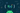### Functional Programming in Kotlin - f(2)

Functions in Kotlin | Pure Functions

May 3rd, 2020 · 3 min read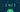### Functional Programming in Kotlin - f(1)

Introduction to Functional Programming in Kotlin

April 24th, 2020 · 5 min read